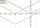# Combinations

If the number of elements increase by 3, it increases the number of combinations of the second class of these elements 5 times. How many are the elements?

Result

n =  3

#### Solution:Checkout calculation with our calculator of quadratic equations.

Leave us a comment of example and its solution (i.e. if it is still somewhat unclear...):Be the first to comment!#### To solve this example are needed these knowledge from mathematics:

Looking for help with calculating roots of a quadratic equation? Would you like to compute count of combinations? Do you have a linear equation or system of equations and looking for its solution? Or do you have quadratic equation?

## Next similar examples:

1. CombinationsHow many elements can form six times more combinations fourth class than combination of the second class?
2. 2nd class combinationsFrom how many elements you can create 4560 combinations of the second class?
3. CombinationsFrom how many elements we can create 990 combinations 2nd class without repeating?
4. Theorem proveWe want to prove the sentence: If the natural number n is divisible by six, then n is divisible by three. From what assumption we started?
5. TrinityHow many different triads can be selected from the group 43 students?
6. Calculation of CNCalculate: ?
7. Fish tankA fish tank at a pet store has 8 zebra fish. In how many different ways can George choose 2 zebra fish to buy?
8. Equation with abs valueHow many solutions has the equation ? in the real numbers?
9. EquationEquation ? has one root x1 = 8. Determine the coefficient b and the second root x2.
10. DiscriminantDetermine the discriminant of the equation: ?
11. RootsDetermine the quadratic equation absolute coefficient q, that the equation has a real double root and the root x calculate: ?Find the roots of the quadratic equation: 3x2-4x + (-4) = 0.If a2-3a+1=0, find (i)a2+1/a2 (ii) a3+1/a3Solve quadratic equation: (6n+1) (4n-1) = 3n2If the square root of 3m2 +22 and -x = 0, and x=7, what is m?solve equation: ?X+y=5, find xy (find the product of x and y if x+y = 5)